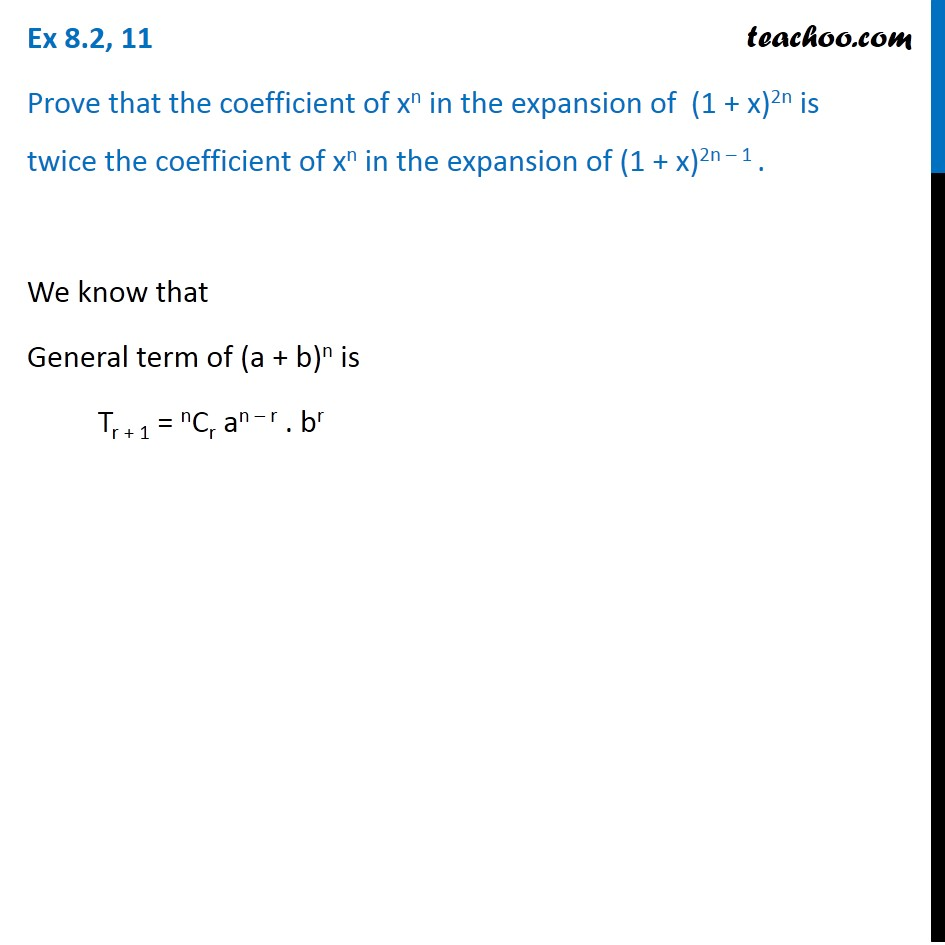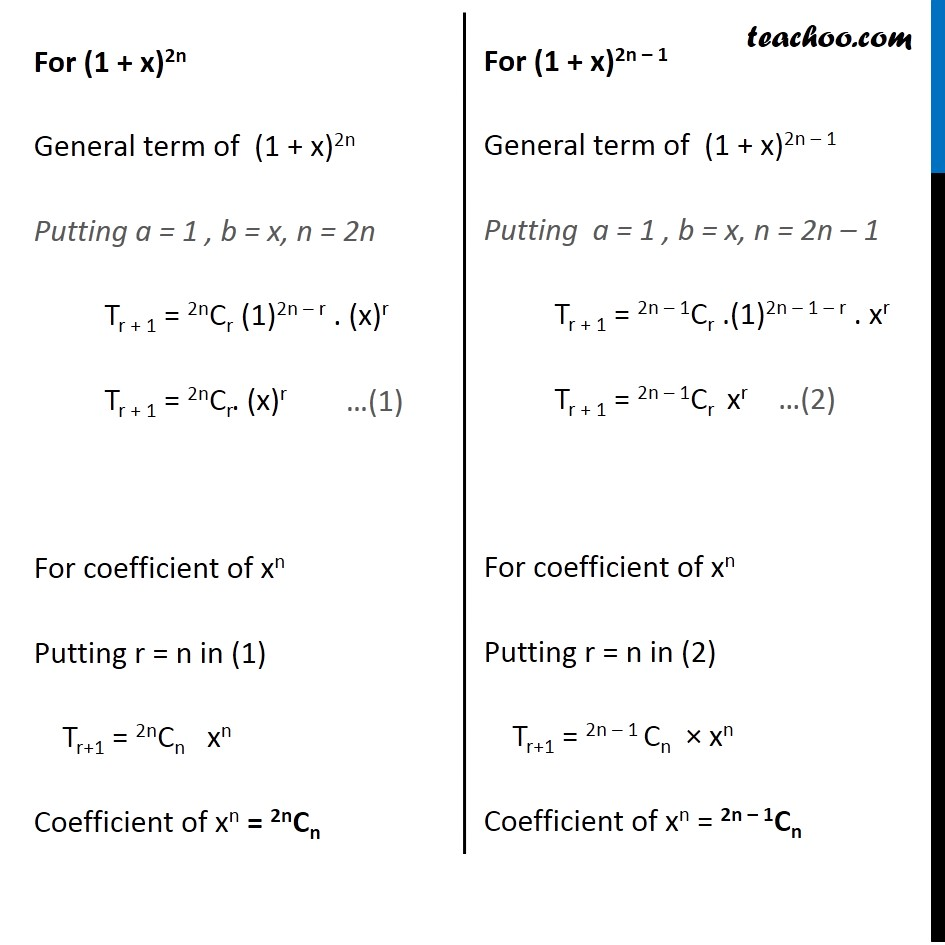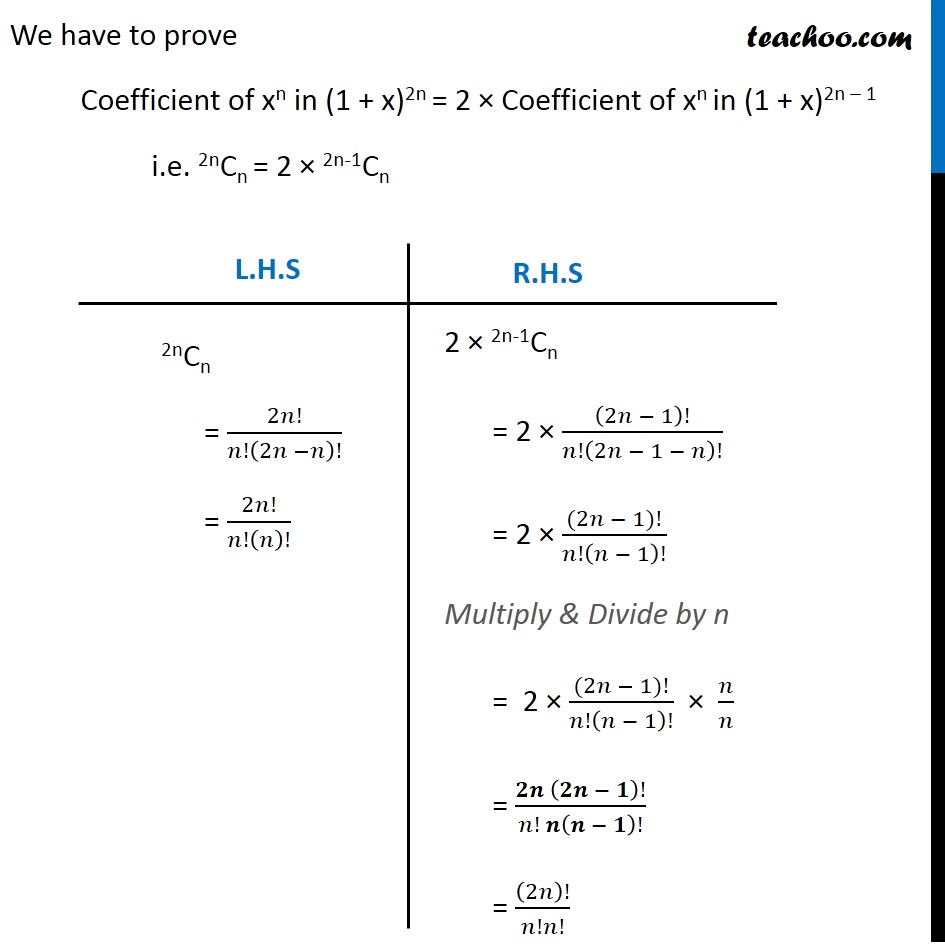General Term of Binomial Theorem

Chapter 7 Class 11 Binomial Theorem
Serial order wiseLearn in your speed, with individual attention - Teachoo Maths 1-on-1 Class

### Transcript

Question 11 Prove that the coefficient of xn in the expansion of (1 + x)2n is twice the coefficient of xn in the expansion of (1 + x)2n – 1 . We know that General term of (a + b)n is Tr + 1 = nCr an – r . br For (1 + x)2n General term of (1 + x)2n Putting a = 1 , b = x, n = 2n Tr + 1 = 2nCr (1)2n – r . (x)r Tr + 1 = 2nCr. (x)r For coefficient of xn Putting r = n in (1) Tr+1 = 2nCn xn Coefficient of xn = 2nCn For (1 + x)2n – 1 General term of (1 + x)2n – 1 Putting a = 1 , b = x, n = 2n – 1 Tr + 1 = 2n – 1Cr .(1)2n – 1 – r . xr Tr + 1 = 2n – 1Cr xr For coefficient of xn Putting r = n in (2) Tr+1 = 2n – 1 Cn × xn Coefficient of xn = 2n – 1Cn We have to prove Coefficient of xn in (1 + x)2n = 2 × Coefficient of xn in (1 + x)2n – 1 i.e. 2nCn = 2 × 2n-1Cn 2nCn = 2𝑛!/𝑛!(2𝑛 −𝑛)! = 2𝑛!/𝑛!(𝑛)! 2 × 2n-1Cn = 2 × (2𝑛 − 1)!/𝑛!(2𝑛 − 1 − 𝑛)! = 2 × ((2𝑛 − 1)!)/𝑛!(𝑛 − 1)! Multiply & Divide by n = 2 × ((2𝑛 − 1)!)/𝑛!(𝑛 − 1)! × 𝑛/𝑛 = (𝟐𝒏 (𝟐𝒏 − 𝟏)!)/(𝑛! 𝒏(𝒏 − 𝟏)!) = (2𝑛)!/𝑛!𝑛! Hence L.H.S = R.H.S Hence Proved